Custom SearchPERMUTATIONS Permutations are similar to combinations but extend the requirements of combinations by considering order.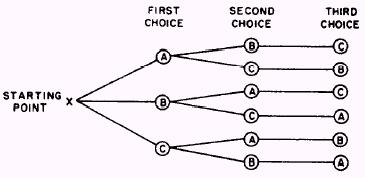Figure 8-1.-"Tree" diagram. Suppose we have two letters, A and B, and wish to know how many arrangements of these letters can be made. Obviously the answer is two; that is, AB and BA If we extend this to the three letters A, B, and C, we find the answer to be ABC, ACB, BAC, BCA, CAB, CBA We had three choices for the first letter; after we chose the first letter, we had only two choices for the second letter; and after the second letter, we had only one choice. This is shown in the "tree" diagram in figure 8-l. Notice that a total of six different paths lead to the ends of the "branches" of the "tree" diagram. If the number of objects is large, the tree would become very complicated; therefore, we approach the problem in another manner, using parentheses to show the possible choices. Suppose we were to arrange six objects in as many different orders as possible. For the first choice we have six objects: STARTING POINT (6)( )( )( )( )( ) For the second choice we have only five choices: (6)(5)( )( )( )( ) For the third choice we have only four choices: (6)(5)(4)( )( )( ) This may be continued as follows: (6)(5)(4)(3)(2)(1) By applying the principle of choice, we find the total possible ways of arranging the objects to be the product of the individiual choices; that is, 6.5.4.3.2.1 and this may be written as 6! This leads to the statement: The number of permutations of n objects taken all together is equal to n!. EXAMPLE: How many permutations of seven different letters may be made? SOLUTION: We could use a "tree" diagram, but this would become complicated. (Try it to see why.) We could use the parentheses as follows: (7)(6)(5)(4)(3)(2)(1) = 5040 The easiest solution is to use the previous statement and write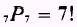that is, the number of possible arrangements of seven objects taken seven at a time is 71. NOTE: Compare this with the number of combinations of seven objects taken seven at a time. If we are faced with finding the number of permutations of seven objects taken three at a time, we use three parentheses as follows: In the first position we have a choice of seven objects: In the second position we have a choice of six objects: (7)(6)( ) In the last position we have a choice of five objects: (7)(6)(5) Therefore by principle of choice, the solution is 7.6.5 = 210 At this point we will use our knowledge of combinations to develop a formula for the number of permutations of n objects taken r at a time. Suppose we wish to find the number of permutations of five things taken three at a time. We first determine the number of groups of three as follows:Thus, we have 10 groups of 3 objects each. We are now asked to arrange each of these 10 groups in as many orders as possible. We know that the number of permutations of three objects taken together is 3!. We may arrange each of the 10 groups in 3! or 6 ways. The total number of possible permutations of 5C3 then is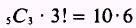which can be written as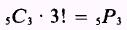The corresponding general form isKnowing thatthenBut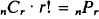therefore,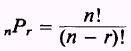This formula is read: The number of permutations of n objects taken r at a time is equal to n factorial divided by n minus r factorial. EXAMPLE: How many permutation of six objects taken two at a time can be made? SOLUTION: The number of permutations of six objects taken two at a time is written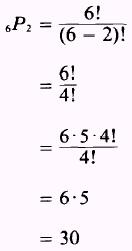EXAMPLE: In how many ways can eight people be arranged in a row? SOLUTION: All eight people must be in the row; therefore, we want the number of permutations of eight people taken eight at a time, which is(remember that 0! was defined as equal to 1) thenProblems dealing with combinations and permutations often require the use of both formulas to solve one problem. EXAMPLE: Eight first class and six second class petty officers are on the board of the 56 club. In how many ways can the members elect, from the board, a president, a vice-president, a secretary, and a treasurer if the president and secretary must be first class petty officers and the vice-president and treasurer must be second class petty officers? SOLUTION: Since two of the eight first class petty officers are to fill two different offices, we write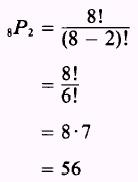Then, two of the six second class petty officers are to fill two different offices; thus, we write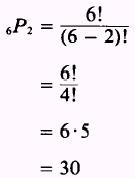The principle of choice holds in this case; therefore, the members have 56.30 = 1,680 ways to select the required office holders. EXAMPLE: For the preceding example, suppose we are asked the following: In how many ways can the members elect the office holders from the board if two of the office holders must be first class petty officers and two of the office holders must be second class petty officers? SOLUTION: We have already determined how many ways eight things may be taken two at a time, how many ways six things may be taken two at a time, and how many ways they may be taken together; that is,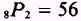and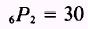then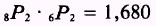Our problem now is to find how many ways the members can combine the four offices two at a time. Therefore, we write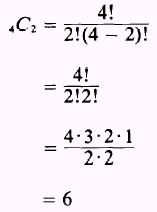Then, in answer to the problem, we writeIn other words, if the members have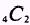ways of combining the four offices and then for every one of these ways, the members have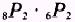ways of arranging the office holders, then they haveways of electing the petty officers.Integrated Publishing, Inc. - A (SDVOSB) Service Disabled Veteran Owned Small Business